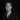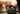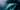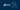Navigate back to the homepage

# Levenshtein's Distance EquationNicolai
April 1st, 2020 · 3 min readRecently, I was working with DGraph to build a knowledge graph. The idea was to create a search engine, that helps to identify which technologies are used across different customers. When reading further through the tutorials, I stumbled upon Levenshtein’s distance equation in the context of fuzzy search.

Providing search capabilities on technologies requires searching for the closest match to a string, if a full match doesn’t exist. This feature helps to get relevant results even if there’s a typo or the user doesn’t search based on the exact name it is stored. This is exactly what fuzzy search does — it compares the string values and returns the nearest matches. Hence, it’s ideal for the use case of implementing a search engine on technologies.

## Levenshtein’s Distance Equation

Here, Levenshtein’s distance is often used as a metric to measure the closeness of two strings. The higher the number, the more operations (insert, substitute, delete) it takes to transform one string into the other. This metric can be calculated with Levenshtein’s distance equation, which is defined as:

### Introduction

For example, the Levenshtein distance between kitten and sitting is 3, because it needs at least 3 edits to change one string into the other.

1. kitten → sitten (substitution of s for k)
2. sitten → sittin (substitution of i for e)
3. sittin → sitting (insertion of g at the end).

Before going into detail and understand Levenshtein’s distance equation, let’s have a quick refresher on functions first.

### Functions

Functions take a given input, follow a set of instructions, and yield an output. A simple linear function may be defined as

which in human words states: Given an input (represented by ), multiply the input by 2 and add 8 to obtain the output.

 input set of instructions output

### Piecewise Functions

Piecewise functions are more complex functions, as there are multiple sets of instructions. One set is chosen over another based on a certain condition. Consider the following example

which in human words states: Given an input (represented by ) …

• If the input is less than 4, square the input
• If the input is equal to 4, the output is 8
• If the input is between 4 and 16, subtract the input from 16
 input set of instructions output

With that in mind, Levenshtein’s distance equation should look a little more readable.

### Levenshtein’s Distance Equation in Detail

Up to this point a basic understanding of functions has been gained and a detailed example can now be discussed. In order to do so, let’s have a look at the parameters first:

• a — 1st string
• b — 2nd string
• i — Terminal character position of 1st string
• j — Terminal character position of 2nd string

An important thing to note is, that the strings are 1-indexed. That being said, the first line of Levenshtein’s distance equation

translates into: If both strings are empty, then the Levenshtein distance equals to 0.

If that’s not the case, then consider the strings pen and pin as example. The numbers in the table below refer to the terminal character position and a and b to the strings to compare.

 1 2 3 a P E N b P I N

To proceed, let’s check each character individually by looping over the terminal positions. For the given example, this results in:

Overall, 1 edit is required to change pen into pin or vice versa.

#### Calculation using a Matrix

Fortunately, the Levenshtein distance can be calculated by solving a matrix. The bottom right corner will then yield the metric. Let’s begin to fill out the matrix by following the piecewise functions.

Having a closer look at the last instruction reveals, that there’s a conditional statement, , which translates into: If both characters are not equal, then add 1 — else, do not account as there’s no edit required.

From the resulting vector, the minimum value is taken as result. As a consequence, the Levenshtein distance between the first letters of string a and b is 0 as they are equal.

When applying the above logic to the matrix, it’s solved as shown in the table below. The bottom right corner yields the Levenshein distance, which in this case is 1, as exactly 1 edit is required.

 # P E N # 0 1 2 3 P 1 0 1 2 I 2 1 1 2 N 3 2 2 1

Vladimir Levenshtein was a Russian scientist, who is best known for the distance equation named after him. He came up with the algorithm in 1965 and it’s worth mentioning, that according to MIT the algorithm hasn’t improved since then. MIT researchers report that, “in all likelihood, […] the algorithm is as good as it gets”.

### Join the mailing list

High-quality blog posts are like shiny Pokémon - they don't appear often. But when they do, be the first to receive the latest content with the ability to opt-out at anytime.

### More articles from Nicolai### Kubernetes Cheatsheet

This post contains a list of commands and tips, that I use often when working with Kubernetes.

August 18th, 2021 · 2 min read### Kong - Log requests to the console

This post explains, how a request can be logged to the console for debugging purposes when using Kong as API gateway.

June 19th, 2021 · 2 min read
Link to $https://github.com/nicolai92Link to$https://www.linkedin.com/in/nicolai92/Link to $https://medium.com/@nicolai92Link to$https://open.spotify.com/user/0d9i7x6e75vd0th0hnok6vpwf?si=wywquy6CQHOXkrtrsWyA4QLink to $https://twitter.com/nicolai4368Link to$https://www.xing.com/profile/Nicolai_Ernst/cv